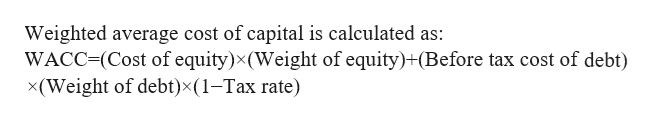# Suppose that JB Cos. has a capital structure of 78 percent equity, 22 percent debt, and that its before-tax cost of debt is 12 percent while its cost of equity is 16 percent. Assume the appropriate weighted-average tax rate is 21 percent and JB estimates that they can make full use of the interest tax shield.What will be JB’s WACC? (Round your answer to 2 decimal places.)WACC: ___.__%

Question
22 views

Suppose that JB Cos. has a capital structure of 78 percent equity, 22 percent debt, and that its before-tax cost of debt is 12 percent while its cost of equity is 16 percent. Assume the appropriate weighted-average tax rate is 21 percent and JB estimates that they can make full use of the interest tax shield.

What will be JB’s WACC? (Round your answer to 2 decimal places.)

WACC: ___.__%

check_circle

Step 1

WACC refers to a firm's weighted average cost of capital. It is the rate that a firm  pays to its security holders in order to finance its assets.

Step 2help_outlineImage TranscriptioncloseWeighted average cost of capital is calculated as: WACC=(Cost of equity)x (Weight of equity)+(Before tax cost of debt) x(Weight of debt)x(1-Tax rate) fullscreen
Step 3

Given that;
Cost of equity is 16%
Weight of equity is 78%
Before tax cost of debt is 12%
Weight of d...

### Want to see the full answer?

See Solution

#### Want to see this answer and more?

Solutions are written by subject experts who are available 24/7. Questions are typically answered within 1 hour.*

See Solution
*Response times may vary by subject and question.
Tagged in